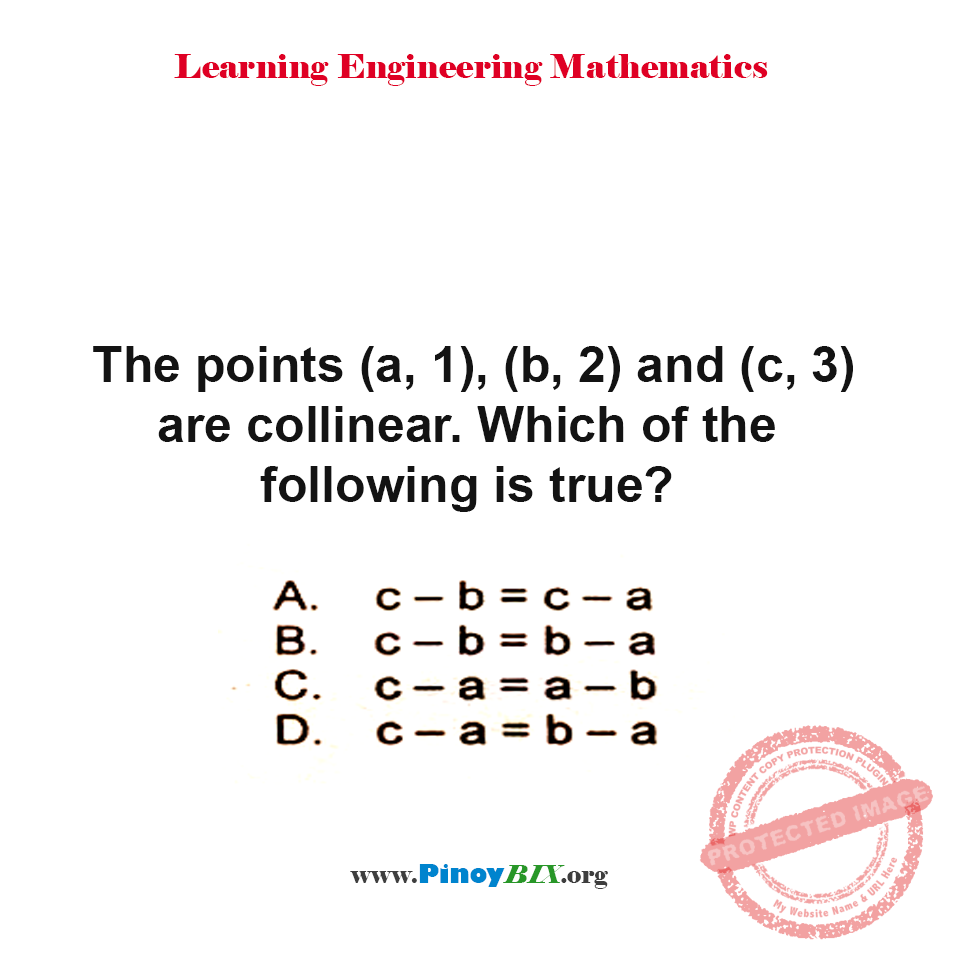Solution: The points (a, 1), (b, 2) and (c, 3) are collinear. Which of the following is true?

(Last Updated On: January 19, 2020)Problem Statement:

The points (a, 1), (b, 2) and (c, 3) are collinear. Which of the following is true?

• A. c – b = c – a
• B. c – b = b – a
• C. c – a = a – b
• D. c – a = b – a

The answer is B. c – b = b – a.

Latest Problem Solving in Analytic Geometry Problems (Points, Lines and Circles)

More Questions in: Analytic Geometry Problems (Points, Lines and Circles)

Online Questions and Answers in Analytic Geometry Problems (Points, Lines and Circles)

1.jackOlGREAAN
PinoyBIX Engineering. © 2014-2021 All Rights Reserved | How to Donate? |